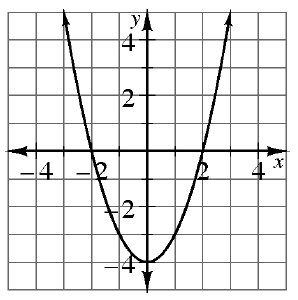### Home > CCG > Chapter 8 > Lesson 8.3.3 > Problem8-120

8-120.

Examine the graph of $f\left(x\right)$ at right. Use the graph to find the following values.

1. $f(1)$

$f\left(1\right)=$ the value of $y$ on the graph when $x = 1$.

$f\left(1\right)=-3$

1. $f(0)$

Locate $x = 0$ on the graph. Find the $y$-coordinate for the point $(0, y)$. This is the value of $f(0)$.

1. $x \text{ if } f(x) = 4$

$f(x) = 4$ is the equivalent of $y = 4$ on the graph.
Therefore, look for the values of $x$ when $y = 4$.

1. $x \text{ if } f(x) = 0$

Find the values of $x$ when $y = 0$, so look for the points $(x, 0)$ on the graph.